# VLOOKUP to compare two columns in Excel for common values and missing data

The tutorial shows how to use VLOOKUP formula in Excel to compare two columns to return common values (matches) or find missing data (differences).

When you have data in two different lists, you may often need to compare them to see what information is missing in one of the lists or what data is present in both. Comparison can be done in many different ways - which method to use depends on exactly what you want from it.

## How to compare two columns in Excel using VLOOKUP

When you have two columns of data and want to find out which data points from one list exist in the other list, you can use the VLOOKUP function to compare the lists for common values.

To build a VLOOKUP formula in its basic form, this is what you need to do:

• For lookup_value (1st argument), use the topmost cell from List 1.
• For table_array (2nd argument), supply the entire List 2.
• For col_index_num (3rd argument), use 1 as there is just one column in the array.
• For range_lookup (4th argument), set FALSE - exact match.

Suppose you have the names of participants in column A (List 1) and the names of those who have passed through the qualification rounds in column B (List 2). You want to compare these 2 lists to determine which participants from Group A made their way to the main event. To do this, use the following formula.

`=VLOOKUP(A2, \$C\$2:\$C\$9, 1, FALSE)`

The formula goes to cell E2, and then you drag it down through as many cells as there are items in List 1.

Please notice that table_array is locked with absolute references (\$C\$2:\$C\$9) so that it remains constant when you copy the formula to the below cells.

As you can see, the names of the qualified athletes show up in column E. For the remaining participants, a #N/A error appears indicating that their names are not available in List 2.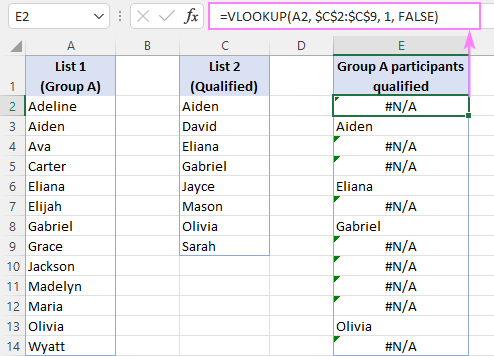### Disguise #N/A errors

The VLOOKUP formula discussed above perfectly fulfills its main objective - returns common values and identifies missing data points. However, it delivers a bunch of #N/A errors, which may confuse inexperienced users making them think that something is wrong with the formula.

To replace errors with blank cells, use VLOOKUP in combination with the IFNA or IFERROR function in this way:

`=IFNA(VLOOKUP(A2, \$C\$2:\$C\$9, 1, FALSE), "")`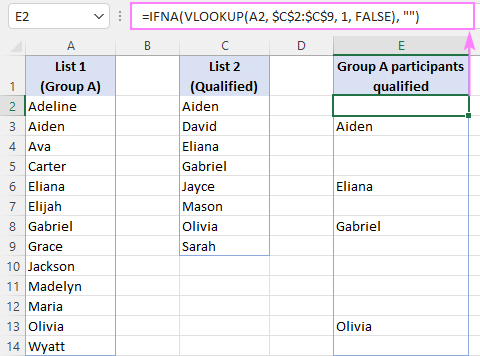Our improved formula returns an empty string ("") instead of #N/A. You can also return your custom text such as "Not in List 2", "Not present", or "Not available". For example:

`=IFNA(VLOOKUP(A2, \$C\$2:\$C\$9, 1, FALSE), "Not in List 2")`

That is the basic VLOOKUP formula to compare two columns in Excel. Depending on your particular task, it can be modified as shown in further examples.

## Compare two columns in different Excel sheets using VLOOKUP

In real life, the columns you need to compare are not always on the same sheet. To search in another worksheet or workbook, you have to use external reference. The best practice is to start typing the formula in your main sheet, then switch to the other worksheet and select the list using the mouse - an appropriate range reference will be added to the formula automatically.

Assuming List 1 is in column A on Sheet1 and list 2 is in column A on Sheet2, you can compare two columns and find matches using this formula:

`=IFNA(VLOOKUP(A2, Sheet2!\$A\$2:\$A\$9, 1, FALSE), "")`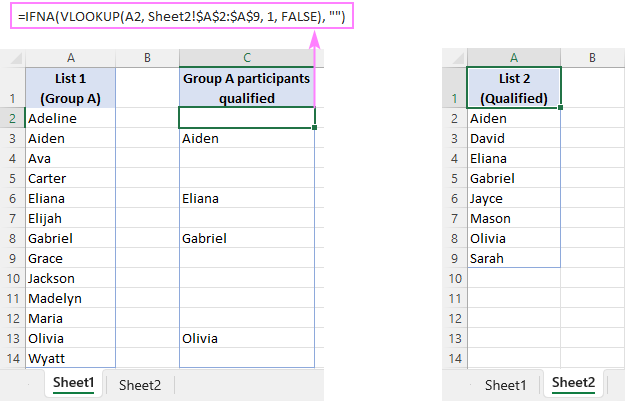## Compare two columns and return common values (matches)

In the previous examples, we discussed a VLOOKUP formula in its simplest form:

`=IFNA(VLOOKUP(A2, \$C\$2:\$C\$9, 1, FALSE), "")`

The result of that formula is a list of values that exist in both columns and blank cells in place of the values not available in the second column.

To get a list of common values without gaps, just add auto-filter to the resulting column and filter out blanks.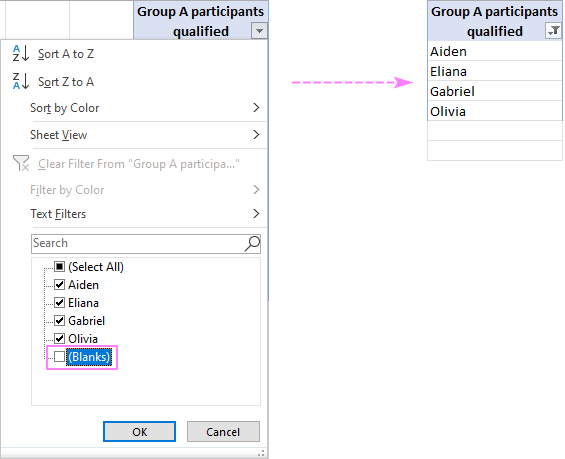In Excel for Microsoft 365 and Excel 2021 that support dynamic arrays, you can make use of the FILTER function to sift out blanks dynamically. For this, utilize the IFNA VLOOKUP formula as the criteria for FILTER:

`=FILTER(A2:A14, IFNA(VLOOKUP(A2:A14, C2:C9, 1, FALSE), "")<>"")`

Please pay attention that in this case we supply the entire List 1 (A2:A14) to the lookup_value argument of VLOOKUP. The function compares each of the lookup values against List 2 (C2:C9) and returns an array of matches and #N/A errors representing missing values. The IFNA function replaces errors with empty strings and serves the results to the FILTER function, which filters out blanks (<>"") and outputs an array of matches as the final result.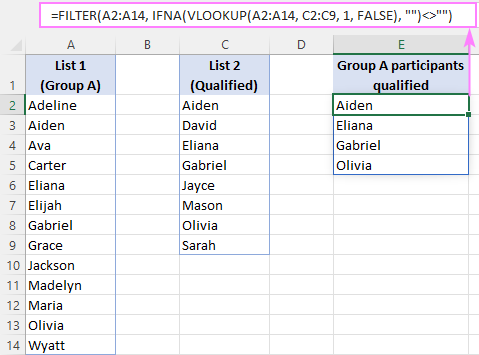Alternatively, you can use the ISNA function to check the result of VLOOKUP and filter the items evaluating to FALSE, i.e. values other than #N/A errors:

`=FILTER(A2:A14, ISNA(VLOOKUP(A2:A14, C2:C9, 1, FALSE))=FALSE)`

The same result can be achieved with the XLOOKUP function, which makes the formula even simpler. Due to the ability of XLOOKUP to handle #N/A errors internally (optional if_not_found argument), we can do without the IFNA or ISNA wrapper:

`=FILTER(A2:A14, XLOOKUP(A2:A14, C2:C9, C2:C9,"")<>"")`

## Compare two columns and find missing values (differences)

To compare 2 columns in Excel to find differences, you can proceed in this way:

1. Write the core formula to search for the first value from List 1 (A2) in List 2 (\$C\$2:\$C\$9):

VLOOKUP(A2, \$C\$2:\$C\$9, 1, FALSE)

2. Nest the above formula in the ISNA function to check the VLOOKUP's output for #N/A errors. In case of an error, ISNA yields TRUE, otherwise FALSE:

ISNA(VLOOKUP(A2, \$C\$2:\$C\$9, 1, FALSE))

3. Use the ISNA VLOOKUP formula for the logical test of the IF function. If the test evaluates to TRUE (#N/A error), return a value from List 1 in the same row. If the test evaluates to FALSE (a match in List 2 is found), return an empty string.

The complete formula takes this form:

`=IF(ISNA(VLOOKUP(A2, \$C\$2:\$C\$9, 1, FALSE)), A2, "")`

To get rid of the blanks, apply Excel's Filter as demonstrated in the above example.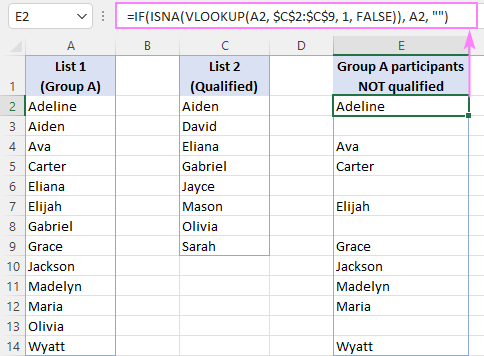In Excel 365 and Excel 2021, you can have the result list filtered dynamically. For this, simply place the ISNA VLOOKUP formula in the include argument of the FILTER function:

`=FILTER(A2:A14, ISNA(VLOOKUP(A2:A14, C2:C9, 1, FALSE)))`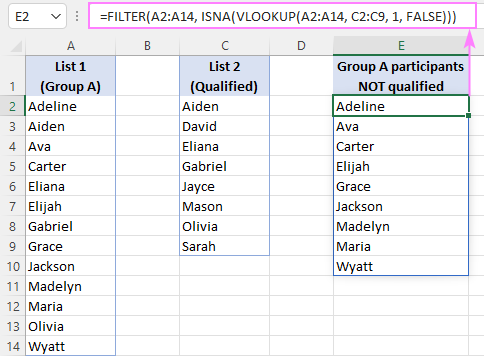Another way is to use XLOOKUP for criteria - the function returns empty strings ("") for missing data points, and you filter the values in List 1 for which XLOOKUP returned empty strings (=""):

`=FILTER(A2:A14, XLOOKUP(A2:A14, C2:C9, C2:C9,"")="")`

## VLOOKUP formula to identify matches and differences between two columns

If you want to add text labels to the first list indicating which values are available in the second list and which are not, use the VLOOKUP formula together with the IF and ISNA/ISERROR functions.

For example, to identify names that are in both columns A and D and those that are only in column A, the formula is:

`=IF(ISNA(VLOOKUP(A2, \$D\$2:\$D\$9, 1, FALSE)), "Not qualified", "Qualified")`

Here, the ISNA function catches the #N/A errors generated by VLOOKUP and passes that intermediate result to the IF function for it to return the specified text for errors and another text for successful lookups.

In this example, we used "Not qualified"/"Qualified" labels, which are suitable for our sample dataset. You can replace them with "Not in List 2"/"In List 2", "Not available"/"Available" or any other labels you see fit.

This formula is best to be inserted in a column adjacent to List 1 and copied through as many cells as there are items in your list.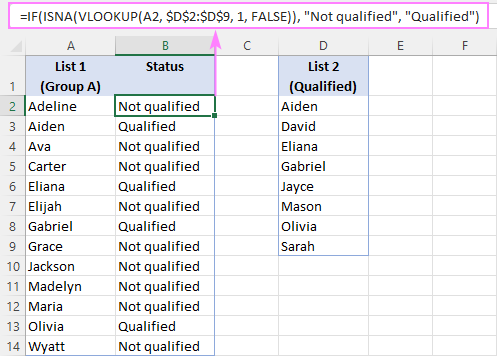One more way to identify matches and differences in 2 columns is using the MATCH function:

`=IF(ISNA(MATCH(A2, \$D\$2:\$D\$9, 0)), "Not in List 2", "In List 2")`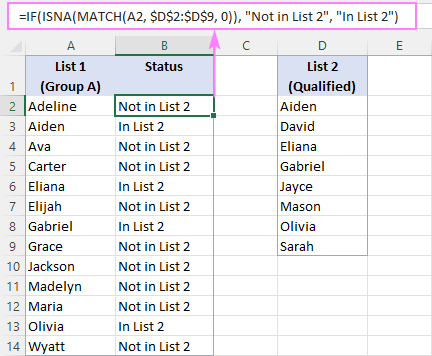## Compare 2 columns and return a value from third

When working with tables containing related data, you may sometimes need to compare two columns in two different tables and return a matching value from another column. In fact, it is the primary use of the VLOOKUP function, the purpose it was designed for.

For example, to compare the names in columns A and D in the two tables below and return a time from column E, the formula is:

`=VLOOKUP(A3, \$D\$3:\$E\$10, 2, FALSE)`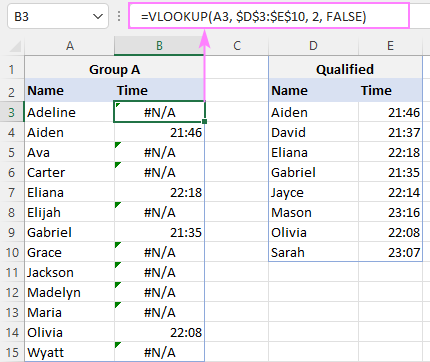To hide #N/A errors, use the proven solution - the IFNA function:

`=IFNA(VLOOKUP(A3, \$D\$3:\$E\$10, 2, FALSE), "")`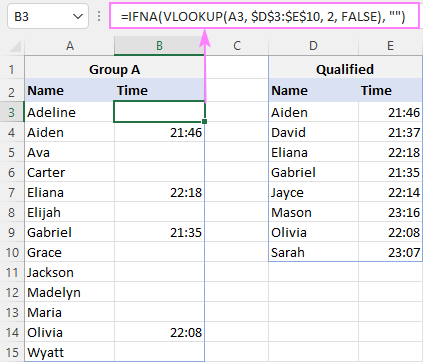Instead of blanks, you can return any text you want for missing data points - just type it in the last argument. For instance:

`=IFNA(VLOOKUP(A3, \$D\$3:\$E\$10, 2, FALSE), "Not available")`

Besides VLOOKUP, the task can be fulfilled with a few other lookup functions.

Personally, I'd rely on a more flexible INDEX MATCH formula:

`=IFNA(INDEX(\$E\$3:\$E\$10, MATCH(A3, \$D\$3:\$D\$10, 0)), "")`

Or use the modern successor of VLOOKUP - the XLOOKUP function, available in Excel 365 and Excel 2021:

`=XLOOKUP(A3, \$D\$3:\$D\$10, \$E\$3:\$E\$10, "")`

To get the names of qualified participants from group A and their results, simply filter out blank cells in column B:

`=FILTER(A3:B15, B3:B15<>"")`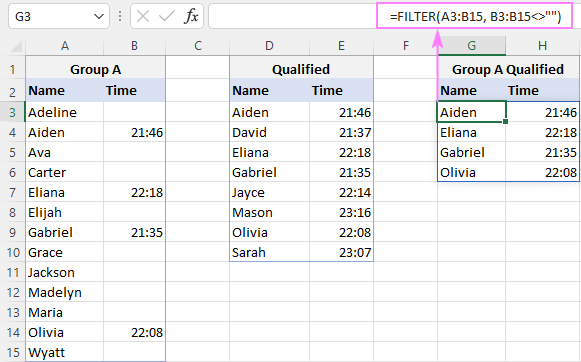## Comparison tools

If you frequently do file or data comparison in Excel, these smart tools included in our Ultimate Suite can save your time immensely!

Compare Tables - quick way to find duplicates (matches) and unique values (differences) in any two data sets such as columns, list or tables.

Compare Two Sheets - find and highlight differences between two worksheets.

Compare Multiple Sheets - find and highlight differences in multiple sheets at once.

VLOOKUP in Excel to compare columns - examples (.xlsx file)

## You may also be interested in

### 14 comments to "VLOOKUP to compare two columns in Excel for common values and missing data"

1.Avinash says:

Hii

In sheet 1, column C (Owner Names) and Column D (Tenant names) are provided along with "Comments" in Column E for a city. And in sheet 2, owner names are provided in column F, and tenant names are in Column H, but comments are not provided.
Now I want, if in sheet1, any of the rows, the Owner name and tenant name exactly match with the owner's name and tenant name in Sheet2 . then the comments should be copied from sheet 1 to sheet 2 in column O. In other words, if Column C & Column D (Sheet1) = Column F & Column H (Sheet 2), then from sheet 1 column E (Comments) should be pasted in Column O in the sheet 2.

2.Ajit says:

=FILTER(A1:A63, ISNA(VLOOKUP(A1:A63, C1:C32, 1, FALSE))) doesn't work. Give a solution that works, dont just post for the sake of posting

A A #SPILL!
B F #SPILL!
C G #SPILL!
D H #SPILL!
E I #SPILL!

•Alexander Trifuntov (Ablebits Team) says:

Hi!
Your VLOOKUP formula returns an array of values. Therefore, a SPILL! error may occur. Read more about this error here: #SPILL! error in Excel - what it means and how to fix.
I don't know what you are trying to achieve with this formula. Therefore, I can not give any advice on how to fix it.

3.Victoria Easton says:

Trying to look for unique values in 2 columns. I have used trim, conditional formatting (unique values) and I am still getting highlighted text even though I can see the same number in the next column!

•Alexander Trifuntov (Ablebits Team) says:

Hello!
I don't see your data. But I assume that your numbers differ by decimal places. If you get numbers using a formula, use round numbers.

4.Vinay says:

Nice!! this worked for me for-

Excellent work guys, keep the good things coming and you would get good blessings in return from individuals like me.

=IFNA(VLOOKUP(A3, \$D\$3:\$E\$10, 2, FALSE), "") :) :)

Thanks,
Vinay S.

5.JdribbleDribble says:

I like to do something like this, I have a table of about 20k items and they are being compared to a new list of items, what i like to do is

if A1 = I1

then copy comment in K1 into G1

the biggest things is that i have some items that are like 8 or 9 all of the same items. I want to ensure they match or skip to the next as it goes through them all.

How is this done without a macro

6.Sazedul Munna says:

Hi
VLOOKUP function I want both whatever in column B & column C and at the same time if any column is empty in column B & column C, need only one cell value. Hope you get my point, waiting for the solution
A B C
ID Class 1 Class 2
1 Q P
2 L
3 R
4 T
5 Y

•Alexander Trifuntov (Ablebits Team) says:

Hi!
Sorry, I do not fully understand the task. Describe in detail what result you want to get.

•Sazedul Munna says:

Hi
In column A there are IDs of some students and in column B & column C these are name of the subjects, vlookup function if I want any ID of a student I need subjects of both if they are in both column B & C. At the same time if any subject is missing in any column these B & C then I need only one subject in vlookup function, hope you get the task. Thanks in advance

Regards
Sazedul Munna

•Alexander Trifuntov (Ablebits Team) says:

Hi!
The information you provided is not enough to understand your case and give you any formula. However, keep in mind that the VLOOKUP function can only return values from one column. To get multiple values using VLOOKUP, use this instruction. You can use two VLOOKUP formulas to get values from two columns.
To get data from multiple columns using a formula, you can use the FILTER function.

•Sazedul Munna says:

Hi
Please advise how can I share my file with you so that I can get my expected result, thanks

Regards
Sazedul Munna